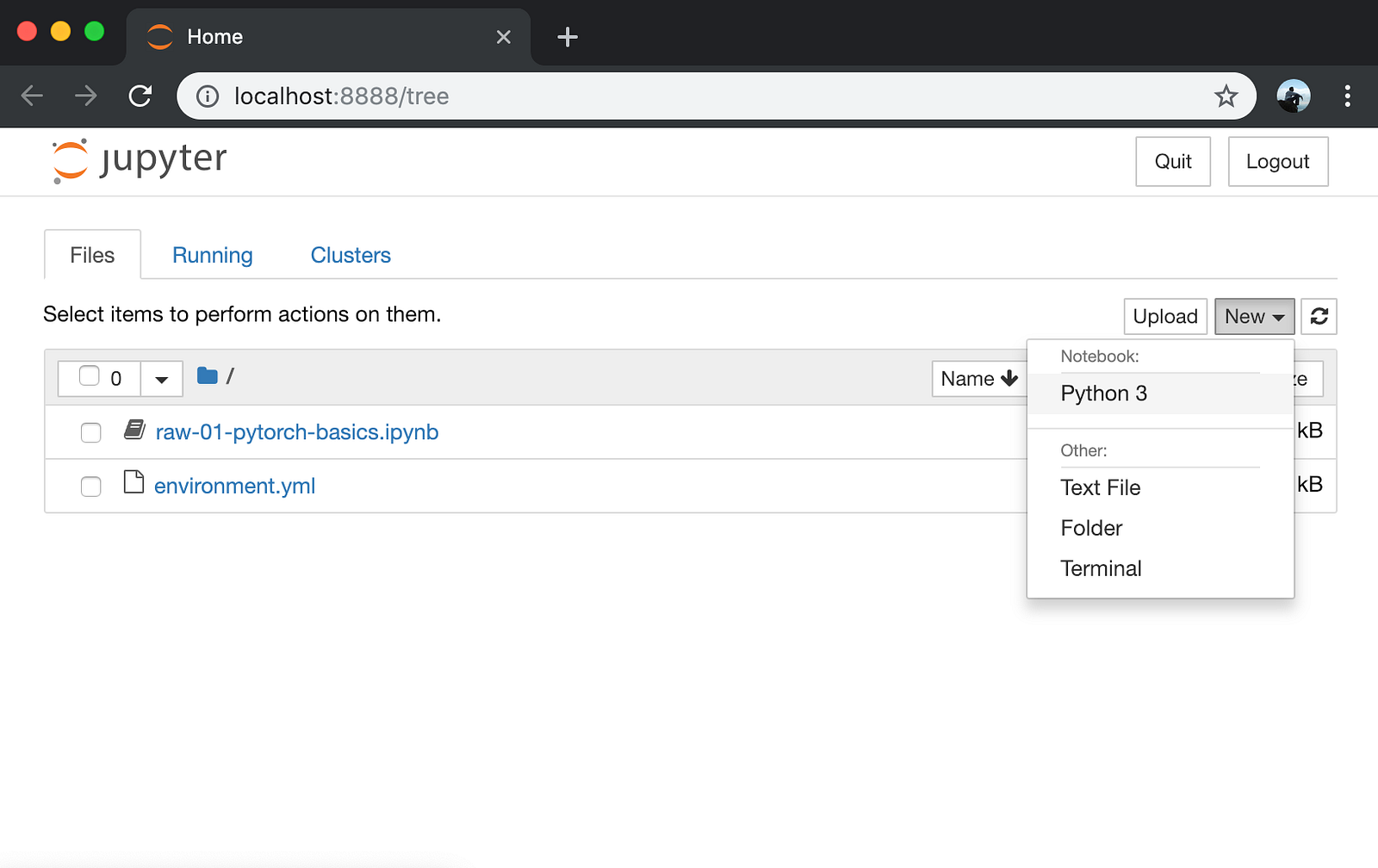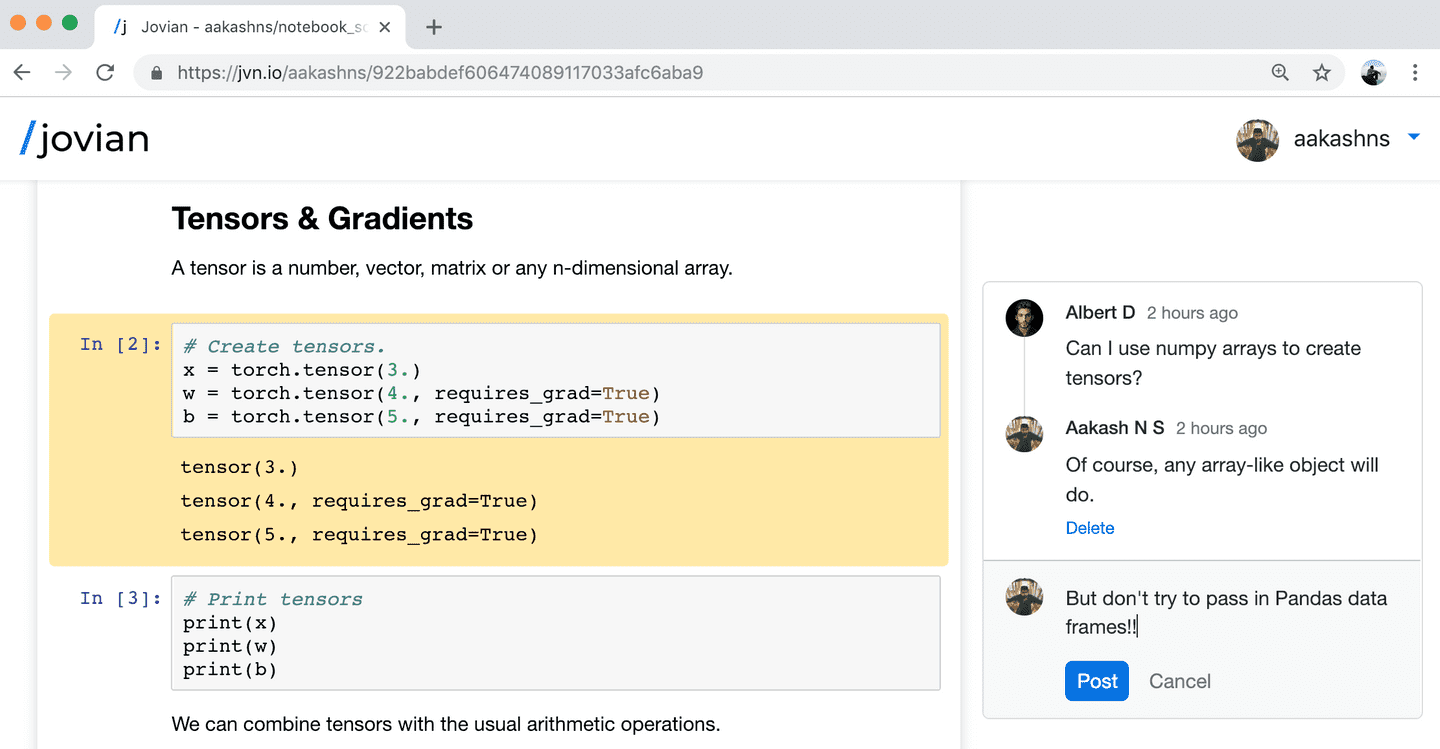## PyTorch Basics: Tensors & Gradients

##### Part 1 of "Pytorch: Zero to GANs"

This post is the first in a series of tutorials on building deep learning models with PyTorch, an open source neural networks library developed and maintained by Facebook. Check out the full series:

1. PyTorch Basics: Tensors & Gradients (this post)
2. Linear Regression & Gradient Descent
3. Image Classfication using Logistic Regression
4. Coming soon.. (neural networks, CNNs, RNNs, GANs etc.)

This series attempts to make PyTorch a bit more approachable for people starting out with deep learning and neural networks. In this post, we’ll cover the basic building blocks of PyTorch models: tensors and gradients.

### System setup

This tutorial takes a code-first approach towards learning PyTorch, and you should try to follow along by running and experimenting with the code yourself. We'll use the Anaconda distribution of Python to install libraries and manage virtual environments. For interactive coding and experimentation, we'll use Jupyter notebooks. All the tutorials in this series are available as Jupyter notebooks hosted on Jovian: a sharing and collaboration platform for Jupyter.

Jovian makes it easy to share Jupyter notebooks on the cloud by running a single command directly within Jupyter. It also captures the Python environment and libraries required to run your notebook, so anyone (including you) can reproduce your work.

Here's what you need to do to get started:

1. Install Anaconda by following the instructions given here. You might also need to add Anaconda binaries to your system PATH to be able to run the `conda` command line tool.

2. Install the `jovian` Python library by the running the following command (without the `\$`) on your Mac/Linux terminal or Windows command prompt:

``````pip install jovian --upgrade
``````
1. Download the notebook for this tutorial using the `jovian clone` command:
``````\$ jovian clone <notebook_id>
``````

(You can get the `notebook_id` by clicking the 'Clone' button at the top of this page on https://jvn.io)

Running the clone command creates a directory `01-pytorch-basics` containing a Jupyter notebook and an Anaconda environment file.

``````\$ ls 01-pytorch-basics
01-pytorch-basics.ipynb  environment.yml
``````
1. Now we can enter the directory and install the required Python libraries (Jupyter, PyTorch etc.) with a single command using `jovian`:
``````\$ cd 01-pytorch-basics
\$ jovian install
``````

`jovian` reads the `environment.yml` file, identifies the right dependencies for your operating system, creates a virtual environment with the given name (`01-pytorch-basics` by default) and installs all the required libraries inside the environment, to avoid modifying your system-wide installation of Python. It uses `conda` internally. If you face issues with `jovian install`, try running `conda env update` instead.

1. We can activate the virtual environment by running
``````\$ conda activate 01-pytorch-basics
``````

For older installations of `conda`, you might need to run the command: `source activate 01-pytorch-basics`.

1. Once the virtual environment is active, we can start Jupyter by running
``````\$ jupyter notebook
``````
1. You can now access Jupyter's web interface by clicking the link that shows up on the terminal or by visiting http://localhost:8888 on your browser.At this point, you can click on the notebook `01-pytorch-basics.ipynb` to open it and run the code. If you want to type out the code yourself, you can also create a new notebook using the 'New' button.

We begin by importing PyTorch:

In :
``import torch``

### Tensors

At its core, PyTorch is a library for processing tensors. A tensor is a number, vector, matrix or any n-dimensional array. Let's create a tensor with a single number:

In :
``````# Number
t1 = torch.tensor(4.)
t1
``````
Out:
``tensor(4.)``

`4.` is a shorthand for `4.0`. It is used to indicate to Python (and PyTorch) that you want to create a floating point number. We can verify this by checking the `dtype` attribute of our tensor:

In :
``t1.dtype``
Out:
``torch.float32``

Let's try creating slightly more complex tensors:

In :
``````# Vector
t2 = torch.tensor([1, 2, 3])
t2
``````
Out:
``tensor([1, 2, 3])``
In :
``````# Matrix
t3 = torch.tensor([[1, 2, 3],[ 3, 5, 8]])
t3
``````
Out:
``````tensor([[1, 2, 3],
[3, 5, 8]])``````
In :
``````# 3-dimensional array
t4 = torch.tensor([[[1, 2], [3, 4]], [[5, 6], [7, 8]]])
t4
``````
Out:
``````tensor([[[1, 2],
[3, 4]],

[[5, 6],
[7, 8]]])``````

Tensors can have any number of dimensions, and different lengths along each dimension. We can inspect the length along each dimension using the `.shape` property of a tensor.

In :
``t1.shape``
Out:
``torch.Size([])``
In :
``t2.shape``
Out:
``torch.Size()``
In :
``t3.shape``
Out:
``torch.Size([2, 3])``
In :
``t4.shape``
Out:
``torch.Size([2, 2, 2])``

### Tensor operations and gradients

We can combine tensors with the usual arithmetic operations. Let's look an example:

In :
``````# Create tensors.
x = torch.tensor(3.)
w = torch.tensor(4., requires_grad=True)
b = torch.tensor(5., requires_grad=True)``````

We've created 3 tensors `x`, `w` and `b`, all numbers. `w` and `b` have an additional parameter `requires_grad` set to `True`. We'll see what it does in just a moment.

Let's create a new tensor `y` by combining these tensors:

In :
``````# Arithmetic operations
y = x * w + b
y
``````
Out:
``tensor(17., grad_fn=<AddBackward0>)``

As expected, `y` is a tensor with the value `3 * 4 + 5 = 17`. What makes PyTorch special is that we can automatically compute the derivative of `y` w.r.t. the tensors that have `requires_grad` set to `True` i.e. w and b. To compute the derivatives, we can call the `.backward` method on our result `y`.

In :
``````# Compute derivatives
y.backward()
``````

The derivates of `y` w.r.t the input tensors are stored in the `.grad` property of the respective tensors.

In :
``````# Display gradients
print('dy/dx:', x.grad)
print('dy/dw:', w.grad)
print('dy/db:', b.grad)``````
```dy/dx: None dy/dw: tensor(3.) dy/db: tensor(1.) ```

As expected, `dy/dw` has the same value as `x` i.e. `3`, and `dy/db` has the value `1`. Note that `x.grad` is `None`, because `x` doesn't have `requires_grad` set to `True`.

The "grad" in `w.grad` stands for gradient, which is another term for derivative, used mainly when dealing with matrices.

### Interoperability with Numpy

Numpy is a popular open source library used for mathematical and scientific computing in Python. It enables efficient operations on large multi-dimensional arrays, and has a large ecosystem of supporting libraries:

• Matplotlib for plotting and visualization
• OpenCV for image and video processing
• Pandas for file I/O and data analysis

Instead of reinventing the wheel, PyTorch interoperates really well with Numpy to leverage its existing ecosystem of tools and libraries.

Here's how we create an array in Numpy:

In :
``````import numpy as np

x = np.array([[1, 2], [3, 4]])
x
``````
Out:
``````array([[1, 2],
[3, 4]])``````

We can convert a Numpy array to a PyTorch tensor using `torch.fron_numpy`.

In :
``````# Convert the numpy array to a torch tensor.
y = torch.tensor(x)
y
``````
Out:
``````tensor([[1, 2],
[3, 4]])``````

Let's verify that the numpy array and torch tensor have similar data types.

In :
``x.dtype, y.dtype``
Out:
``(dtype('int64'), torch.int64)``

We can convert a PyTorch tensor to a Numpy array using the `.numpy` method of a tensor.

In :
``````# Convert a torch tensor to a numpy array
z = y.numpy()
z
``````
Out:
``````array([[1, 2],
[3, 4]])``````

The interoperability between PyTorch and Numpy is really important because most datasets you'll work with will likely be read and preprocessed as Numpy arrays.

### Commit and upload the notebook

As a final step, we can save and commit out work using the `jovian` library.

In :
``import jovian``
In [ ]:
``jovian.commit()``
```[jovian] Saving notebook.. ```

Jovian uploads the notebook to https://jvn.io, captures the Python environment and creates a sharable link for your notebook as shown above. You can use this link to share your work and let anyone reproduce it easily with the `jovian clone` command. Jovian also includes a powerful commenting interface, so you (and others) can discuss & comment on specific parts of your notebook:### Further Reading

Tensors in PyTorch support a variety of operations, and what we've covered here is by no means exhaustive. You can learn more about tensors and tensor operations here: https://pytorch.org/docs/stable/tensors.html

You can take advantage of the interactive Jupyter environment to experiment with tensors and try different combinations of operations discussed above. Here are some things to try out:

1. What if one or more `x`, `w` or `b` were matrices, instead of numbers, in the above example? What would the result `y` and the gradients `w.grad` and `b.grad` look like in this case?

2. What if `y` was a matrix created using `torch.tensor`, with each element of the matrix expressed as a combination of numeric tensors `x`, `w` and `b`?

3. What if we had a chain of operations instead of just one i.e. `y = x * w + b`, `z = l * y + m`, `w = c * z + d` and so on? What would calling `w.grad` do?

If you're interested, you can learn more about matrix derivates on Wikipedia (although it's not necessary for following along with this series of tutorials): https://en.wikipedia.org/wiki/Matrix_calculus#Derivatives_with_matrices

With this, we complete our discussion of tensors and gradients in PyTorch, and we're ready to move on to the next topic: Linear regression.

### Credits

The material in this series is heavily inspired by the following resources:

1. PyTorch Tutorial for Deep Learning Researchers by Yunjey Choi:

2. FastAI development notebooks by Jeremy Howard: